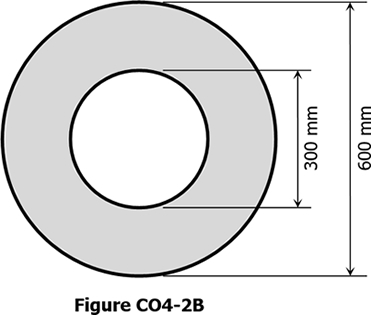# circular beam

Situation
A concrete beam with cross section in Figure CO4-2B is simply supported over a span of 4 m. The cracking moment of the beam is 75 kN·m.1.   Find the maximum uniform load that the beam can carry without causing the concrete to crack, in kN/m.

 A.   35.2 C.   33.3 B.   37.5 D.   41.8

2.   Find the modulus of rapture of the concrete used in the beam.

 A.   4.12 MPa C.   3.77 MPa B.   3.25 MPa D.   3.54 MPa

3.   If the hallow portion is replaced with a square section of side 300 mm, what is the cracking moment of the new section in kN·m?

 A.   71.51 C.   78.69 B.   76.58 D.   81.11

## Bending Stress and Shearing Stress in Timber Beam

 Bending Stress $f_b = \dfrac{M}{S} = \dfrac{Mc}{I}$   Horizontal Shear Stress $f_v = \dfrac{VQ}{Ib}$For Rectangular Sections $f_b = \dfrac{6M}{bd^2}$   $f_v = \dfrac{3V}{2bd}$